# (straight) line

## line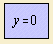Let's have a rectangular coordinate system, built of a two straight lines, the horizontal x-axis (the abscissa) and the vertical y-axis (the ordinate). Given two points A and B, a straight line through these points makes the shortest path between A and B. The angle of the line with a coordinate axis is constant, and also the derivative of (a function represented by) a straight line is constant.
The straight line must be one of the earliest curves studied. Euclid studied the line in his 'Elements', but he did not consider the line being a curve.
An old name for the line is the right line 1).

When the center of inversion is not on the line, the inverse of the line is a circle.
And the line is the pedal of the parabola.

The straight line stands for a linear relation, a linear relationship between two variables. In nature there are a lot of phenomena which are related in a linear way. An example is that the relative stretching of a bar grows linearly with the exerted force.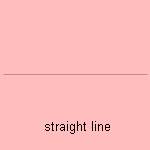A lot of straight lines have been given special names, because of their relation to other curves or figures (as a circle or a triangle). A straight line that touches a curve is called a tangent. When this occurs in infinity, the curve is called an asymptote 2). The line is the only curve for which its tangent coincides with the curve itself.

A bitangent to a curve is a line that touches the curve in two distinct points, where the tangents have the same direction at these points. A consequence of Bézout's theorem is that a plane curve with a bitangent must have a degree of 4 or higher.

A chord is the line between two points on a circle.

In geometry much attention has been given to the triangle. A side of a triangle is called a leg; a special case is the slant side of a right-angled triangle: the hypotenuse. While exploring its qualities, many auxiliary lines has been defined. The perpendicular is dropped down from a vertex perpendicular to the opposite side. In Dutch, each raised perpendicular is called a 'loodlijn' (plumb-line or lead-line), which use goes back to the Middle Ages, when the plumb-line from a boat was used to gauge the depth of the water.
The perpendicular bisector is a perpendicular on the middle of a line segment.
The median directs from a vertex to the opposite side's mid 3). The bisector divides the angle in two (equal) parts, the trisector in three equal parts. A bit far-fetched is the symmedian, the reflection of the median in a bisector. Euler proved that - in a triangle - the points of intersection of the perpendicular bisectors, the perpendiculars, and the medians lie on a line: Euler's line.

A line of Pascal is formed the the cuttings of the opposites of hexagon inscribed in a conic section.
Given six vertices there are 60 Pascal lines.
These lines form an interesting pattern. For a circle the lines are the following, for subsequent zooming out with a factor 2: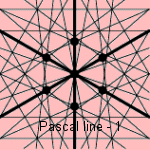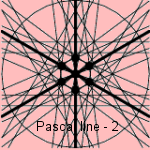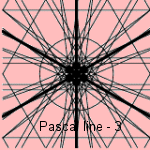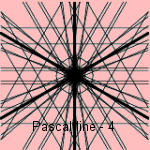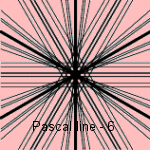You can only see 45 lines, because the three thick lines represent a degenerate group of four Pascal lines, and six of the Pascal lines lie in infinity.
The 60 Pascal lines intersect three at a time in 20 Steiner points, and also in 60 Kirkman points. Each Steiner point lines together with three Kirkman points on 20 Cayley lines.

In a polygon a diagonal is a line between vertices that are no neighbors.

In a conic section the directrix is the line for which the distance to the focus is a constant.

Special cases of the straight line are the constant function (represented by a horizontal line), and the identical function (line y=x).

The straight line refers to a period, many centuries ago, when measuring (of land) took place with ropes. Herodotus describes 4) that after the inundations of the Nile the Egyptian 'rope-stretchers' fixed again the boundaries of the fields. With ropes. They measured too for the pyramids and temples that were being built. That's why the word 'straight' is related to 'to stretch', and the 'line' to 'linen'.

Thousands year ago the Chinese read the future using (uninterrupted) yang lines (saying yes) and (interrupted) yin lines (saying no). This system developed via a set of two lines (earth and heaven) to a set of three lines (trigram). Each trigram has its each own meaning, for example the trigram 'soen' means soft, tenacious, pervasive: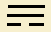. Later two trigrams were combined to the 'hexagram'.

The straight lines that surround us are mainly constructed by Man. In nature we see them as a result of a force directing in one direction: corn grows in the direction of the sun. A stone falls in a straight line down to earth.
Man-made straight lines we see in our houses as ceilings, walls, window-frames, doors. In this way building is possible faster en more standardized. Think about a home with rooms in the form of ellipses or parabolas and all circular windows. Much more work. Or many curlicues on the ceiling, that is not fit to our age. The modern man goes straight to his goal.

Straight is found in many words and expressions, figurative going in a straight line. A Dutch expression as 'recht door zee' ('straight through the sea': be straightforward) sounds more positive than 'recht in de leer' ('straight in the faith': sound in the faith), where a certain amount of stiffness can be heard.
In situations with moving objects (as cars), we see a straight line when a sharp bend can't be made. You have to do something to gain control in a bend, when you don't do anything, you will follow your impulse in a straight line. Physically a straight line is a very natural line. Physics defines a uniform straight motion, when the forces on an object are in equilibrium. But the magnitude of the velocity is relative, it has only meaning in relation to another object.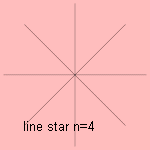A line star is composed of n regular placed equal line pieces.
N = 2 gives a cross.
N = 3 gives the typographic star or asterisk, used for foot-notes: *.
For n = 4 we see the picture to the right.

notes

1) In Dutch a straight line is called a ´rechte´ or a ´rechte lijn´.

2) asumptotos (Lat.) = not meeting.

3) In Dutch the median is called the 'zwaartelijn' (gravity line), a good choice because the two parts in a triangle divided by the median are equal in weight.

4) Historiae II, 109.